## Introduction

An algebraic expression is a mathematical statement containing variables. An algebraic expression can be described as a combination of constants and variables with four mathematical operations: addition, subtraction, multiplication, and/or division.

Rahul is making a pattern using matchsticks for his craftwork. He wants to know how many matchsticks are required to complete the pattern. How does he find that?

Let us find it out in this video.

In this video, we saw

• The need for learning algebraic expressions.
• The relation between known and unknown quantities can be formed using an algebraic expression.

Also, we learnt that the number of matchsticks required can be expressed as an algebraic expression. Similarly, mathematical rules and formulae can be written as algebraic expressions.

For example, the area of a rectangle is calculated as the product of the length and the breadth of the rectangle, i.e., area = length × breadth

We can write this formula as an algebraic expression by considering the length and the breadth of a rectangle as variables namely, ‘l’ and ‘b,’ respectively.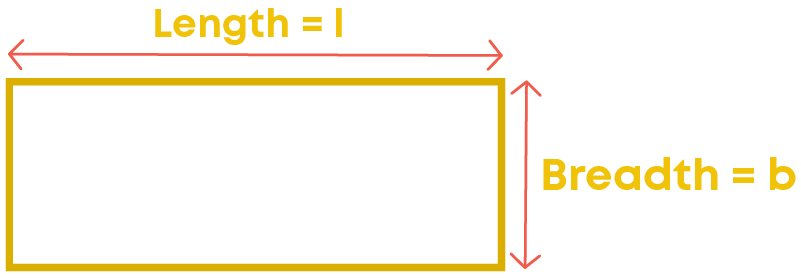So, the formula to find the area of a rectangle can be written as:Area is written as the expression ‘lb’.

Algebraic expressions are used to generalise rules of patterns, rules of geometry etc.

For example,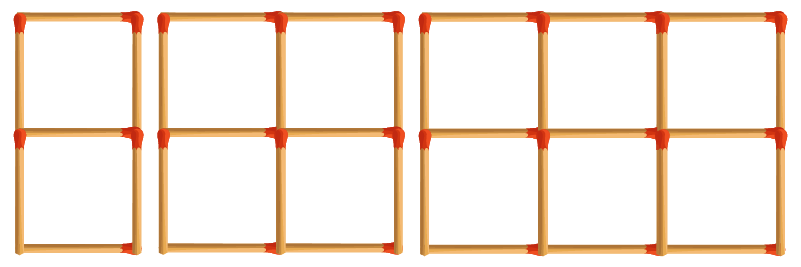To continue the pattern, we should know how many squares will be formed next. How many squares are there in the first set? There are 2. How many are there in the second one? It is 4. In the third one there are 6 squares.

Do you find any common relation between the position of the set of squares and the number of squares in the set?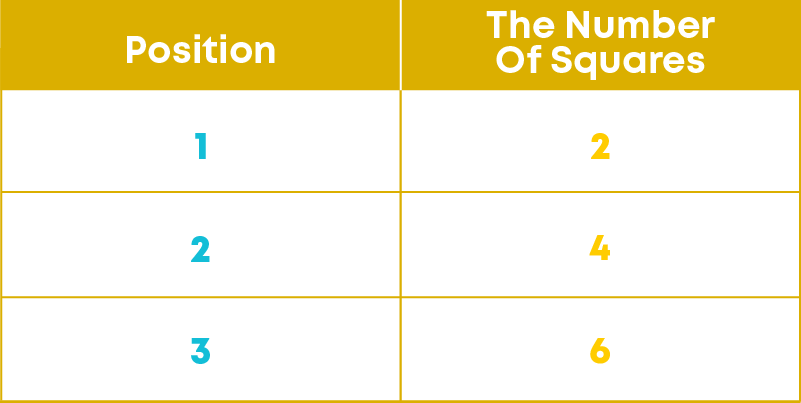Aren’t the numbers in the second column doubles of those in the respective row of the first column?

Yes.

If a set is in the position number ‘n’, what is the number of squares in that set? It is double of n. i.e., 2n.

By considering the position of the pattern as a variable ‘n’, we find the number of squares at the nth place as 2n.

• Algebraic expressions contain variables and constants.
• Variables can take different values at different situations.
• Algebraic expressions help us to represent any rule as a general expression.

#### Concepts

The chapter ‘Algebraic Expressions’ covers the following concepts:

#### Terms Of An Expression

An algebraic expression can be described as a combination of constants and variables with four mathematical operations: addition, subtraction, multiplication, and/or division. Example: 2x + 7 is an algebraic expression. How is this expression obtained?

We see that the variable x is multiplied by 2 and then 7 is added to the result, i.e., 2x is a part of the expression which is formed separately and then another part of the expression 7 is added to it.

Thus, 2x and 7 are parts of the expression which are added to form the expression 2x + 7. These parts of the expression are called its terms.

So, 2x and 7 are the terms of the expression 2x + 7.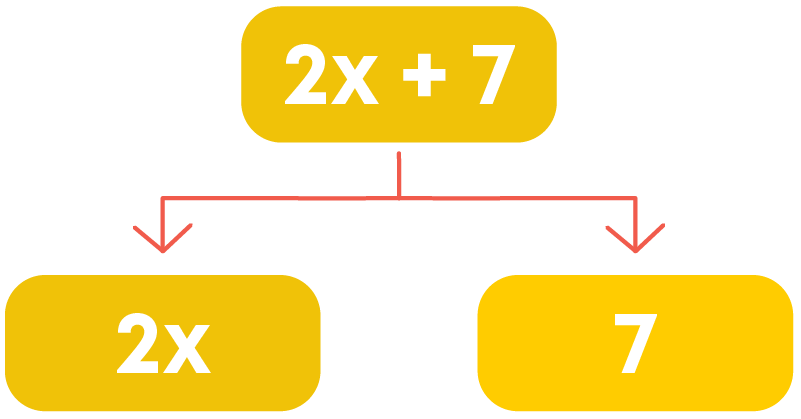A term is a combination of constants and variables with the operation of multiplication or division. A term can be made up of only a variable or only a constant.

For example, in the expression 2x + 7, 7 is a term which is made up of only a constant.

Consider the expression y – 5. y is a term which is made up of only a variable. Which is the other term? Is it 5 or -5?

To identify a term, we should include its sign also.

y – 5 can be written as y + (-5).

Thus, y and -5 are added to give the expression y – 5.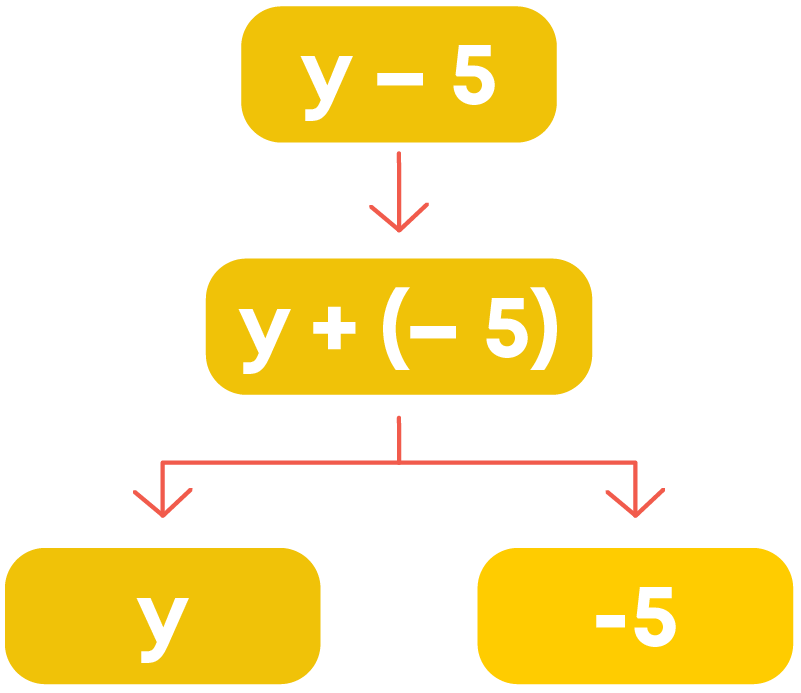• Constants and variables are combined by multiplication/division to form a term
• Different terms are combined by addition to form an algebraic expression.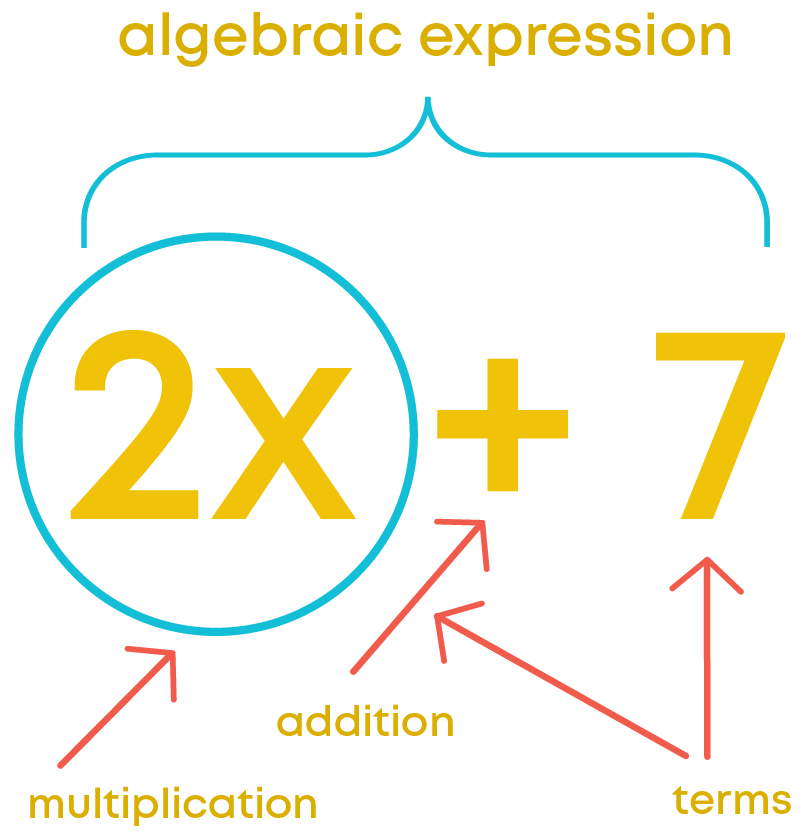Terms of an algebraic expression are parts of the expression added up to give the expression. We know about factors of numbers.

Every number can be written as the product of its factors. For example, 20 can be written as 2 × 10 or 2 × 2 × 5 or 4 × 5. Also, we know that constants and variables combined by multiplication form a term. So, a term also has factors.

How do you write '3yz' as a product of variables and constants?Now, can we identify the factors of the term 3yz? Factors of the term 3yz are 3, y and z. We can show the factors of a term using a factor tree.Does this term have any constant as its factor? Yes, 3 is a constant (number) which is a factor of the term 3yz. So, it is called the numerical factor of the term.

Also, we observe that 3yz has y and z as its factors which are variables. They are called algebraic factors or literal factors of the term.

A number which is a factor of a term is called its numerical factor.

A variable which is a factor of a term is called its algebraic factors or literal factor.

To draw a tree diagram of an algebraic expression we follow these steps:

• Split the expression into its terms.
• Split each term of the expression into its factors.
• If we get factors which can be factorised further, then those factors should be factorised further.
• When we get all the non-factorizable factors, the tree diagram of the expression is complete.

The numerical factor of a term is called the coefficient of the term. It is also called the numerical coefficient of the term.

Consider the term 9a2b.

9a2b = 9 × a × a × b

The factors of the term are 9, a, and b. The numerical factor is 9, also called the coefficient of the term 9a2b. But we have other factors ‘a’ and ‘b’ which are literal factors of the term.

9 is also called the coefficient of a2b.

Similarly,

• ‘a’ is called the coefficient of 9ab
• ‘b’ is called the coefficient of 9a2
• ab is called the coefficient of 9a
• 9b is called the coefficient of a2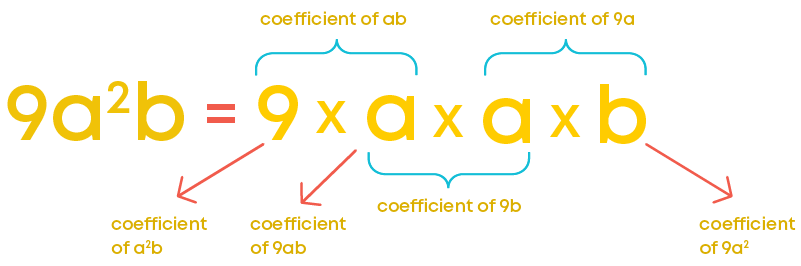Consider another term pq2. What is its coefficient?

We cannot find a number written in the term.

But pq2 can be written as 1 × p × q × q.

That means 1 is its numerical factor.

So, the coefficient or the numerical coefficient of the term pq2 is 1.

Similarly, the coefficient or the numerical coefficient of the term -pq2 is -1. Thus, each factor of a term is called the coefficient of the product of other factors and vice versa.

We find that the terms 2pq and -12pq have the same literal factors. Such terms are called like terms.

Consider the terms 9a2b and 8ab. They have the same literal factors, but they are not like terms. Let us understand why.

9a2b = 9 × a × a × b8ab = 8 × a × bDo you find any difference between the factorisation of the two terms apart from the numerical factors?

Yes, we find that in the factorisation of the term 9a2b, the algebraic factor ‘a’ appears twice but in that of 8ab it appears only once. Or in other words, we can say, the term 9a2b has its literal factor ‘a’ with an exponent 2 but 8ab has its literal factor ‘a’ with an exponent 1. Hence, they are not like terms. Such terms which are not like terms are called unlike terms.

Terms having the same algebraic factors/literal factors/variables with the same power are called like terms. Example: 2x, 3x, -x, -9x, etc.

Terms having different algebraic factors or having algebraic factors with different powers are called unlike terms. Example: 8x, 8xy, -2y, 5a, 3x2, etc.

We have learnt how to identify like terms and unlike terms. Let us consider the algebraic expression 5c2 + 3b – 9c2 + 3bc2

We know that like terms and unlike terms are decided based on the algebraic factors of the terms. Let us use a tree diagram to identify the factors of the terms of an algebraic expression.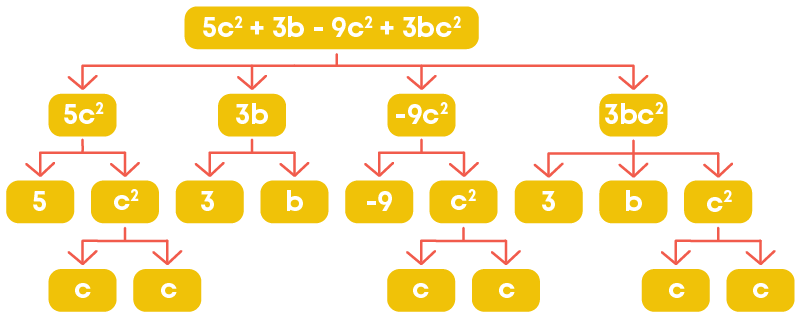We are not bothered about the numerical factors of the terms. We just must check the algebraic factors (variables).

In the tree diagram, we find that the terms 5c2 and -9c2 have the same algebraic factors with the same power.

So, those two terms of the expression are like terms. All other terms are unlike terms.

Thus, to identify like and unlike terms of an algebraic expression,

• Find the factors of the terms
• Check the algebraic factors
• Those terms having the same algebraic factors with the same power are like terms and the other terms are unlike terms.

#### Types Of Polynomials

Different algebraic expressions can have different number of terms. Also, the terms of an algebraic expression can be like terms or unlike terms. Based on these properties algebraic expressions can be classified.

In other words, algebraic expressions can be classified on the number of unlike terms in it.

Consider the term 5t. This is also considered as an algebraic expression.

Any single term can be considered as an algebraic expression with one term. Such expressions are called monomials. The prefix ‘mono’ means one.

How many unlike terms does the expression t2 + 2 have?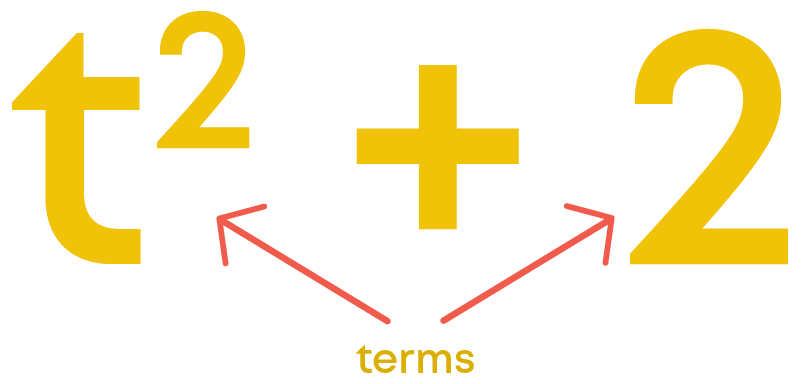It has two unlike terms. Such an algebraic expression is called a binomial. The prefix ‘bi’ means two.

An algebraic expression with three unlike terms is called a trinomial. The prefix ‘tri’ means three.

Example, 3x + 4xy – 8y is a trinomial. Is the expression 3x + 4xy – 8x, a trinomial?

We find two like terms in the expression 3x and -8x. The two like terms in the expression can be simplified to get a single term (we will be learning about that later in the chapter).

So, after simplification, we get only two terms in the expression. Thus, it cannot be a trinomial. Hence, we mention ‘unlike terms’ while defining a binomial and a trinomial.

In general, an expression with one or more terms is called a polynomial.

‘Poly’ means ‘many’, which may refer to any number.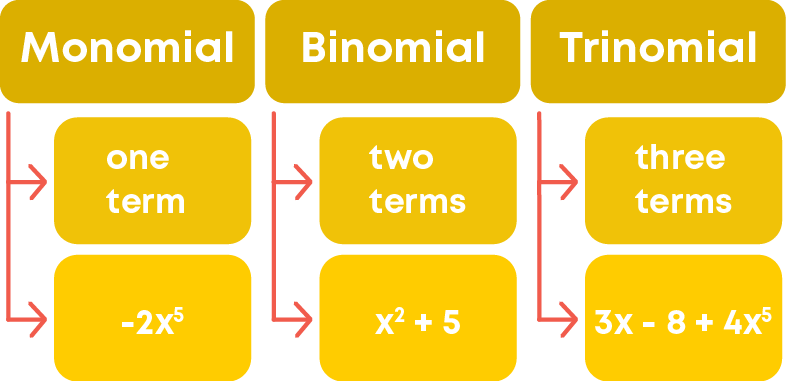We know that polynomials are classified as monomials, binomials and trinomials based on the number of unlike terms in the polynomial. For example, consider the polynomial 3p2q – p + 2

How many terms are there? There are three terms. Do you find any like terms? No, in the given polynomial, there are no like terms.

So, the polynomial 3p2q – p + 2 has three unlike terms. Hence, it is a trinomial.

#### Addition And Subtraction Of Algebraic Terms

Remember these points:

• We can add only like terms.
• When two like terms are added we get a single term as the result.
• When two unlike terms are added we do not get a single term as the result.

Consider the like terms -2y, 5y and y. Let us learn how to add these terms

Write the terms as the products of their factors:What do you observe in the above expansion? Isn’t ‘y’ a common factor of the three terms? Can we use distributive property here? We have already learnt about distributive property of numbers. Can that be applied here? Since variables are also numbers, distributive property can be applied.

On applying distributive property, we get,

[1 + (-2) + 5] × y = 1 × y + (-2) × y + 5 × y.

Hence,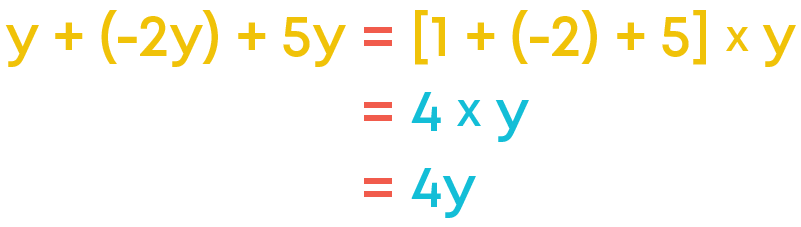The sum of two or more like terms with the numerical coefficient equal to the sum of the numerical coefficients of the given like terms.

We learnt how to add two or more like terms. Using distributive property, we add the numerical coefficients of the like terms, while adding the like terms. Is it applicable to subtraction also? Yes, we have learnt that multiplication is distributive over subtraction also, i.e., for any three numbers a, b, and c,

a (b – c) = ab – ac.

Consider the like terms -2y and 5y. How do you add them?

-2y + 5y = (-2 + 5)y = 3y

Let us add the terms 5x2y and 8x2y: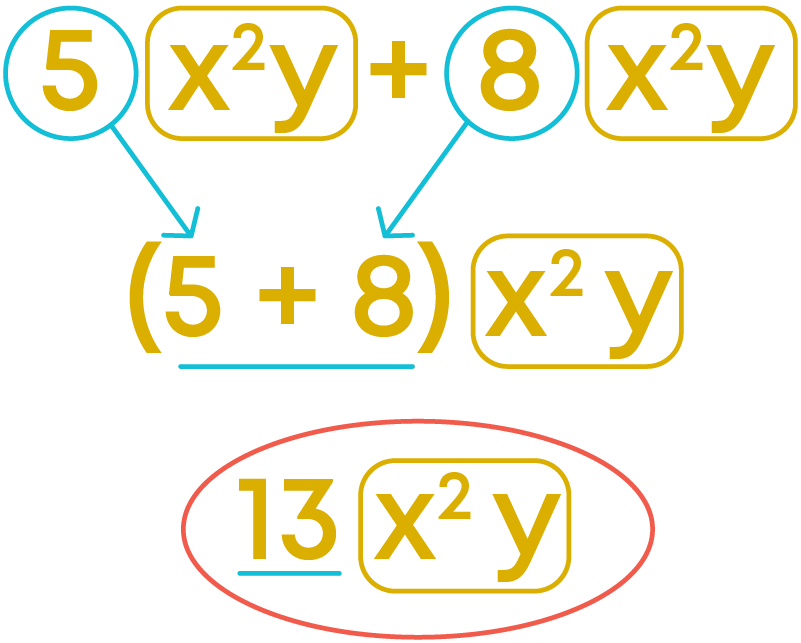Now, let us learn how to subtract one term from the other. As we use distributive property for addition, for subtraction, too, we use distributive property.

Write the terms as the products of their factors.Now, using distributive property we get,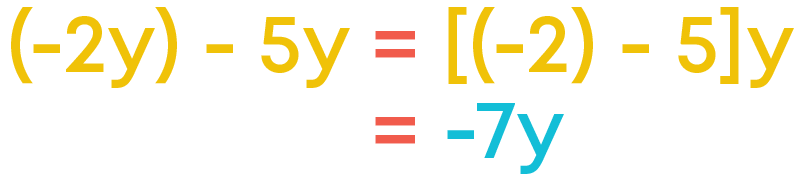The difference of two or more like terms with the numerical coefficient equal to the difference of the numerical coefficients of the given like terms.

Let us subtract the term 2x2y from 10x2y: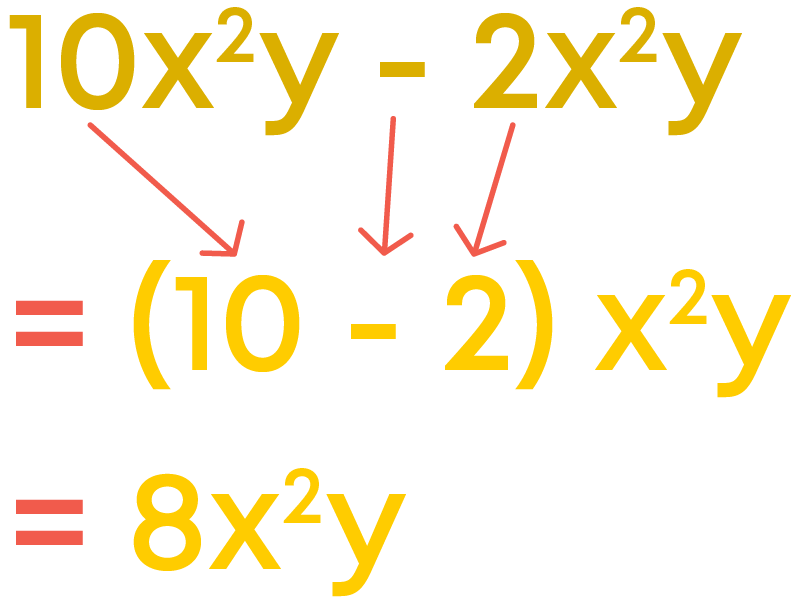#### Addition And Subtraction Of Algebraic Expressions

We know that two or more like terms can be added to give a single term. Similarly, a term can be subtracted from a like term to give a single term. But, unlike terms cannot be added or subtracted the way like terms are. So, when we add or subtract algebraic expressions, only like terms get added/subtracted to give a single term.

For example, consider the algebraic expressions 7x2 – x3 + x and 2x3 – x2. Let us add them.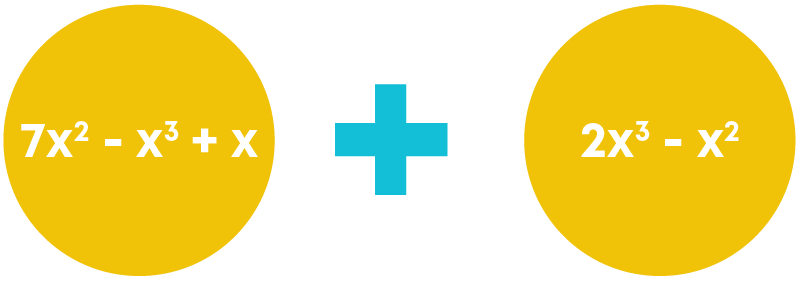Do these expressions have any like terms? Are 7x2 and – x2 like terms?

Yes. How do you add these two terms? Their coefficients will be added. So,

7x2 + (-x2) = [7 + (-1)]x2 = 6x2

Do they have any other like terms?

Yes, -x2 and 2x2 are like terms. So, they can also be added to get a single term. Now, let us add the expressions.

Arrange the like terms together: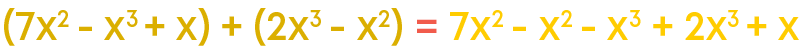Simplify by adding/subtracting the like terms: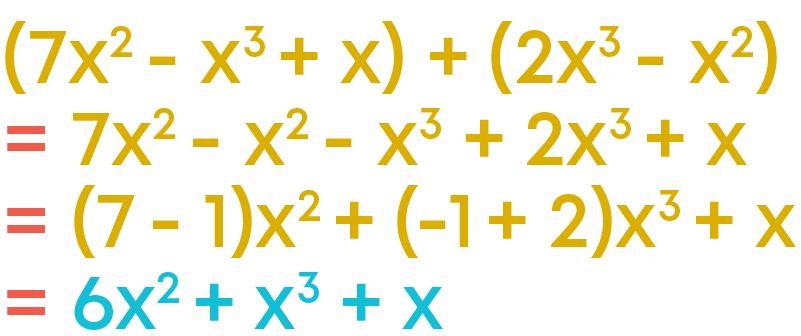• Only like terms can be added/subtracted to give a single term
• Unlike terms will be written as they are.

We know that two or more like terms can be added to give a single term. But, unlike terms cannot be added the way like terms do. So, when we add algebraic expressions, only like terms get added to give a single term. To add two or more algebraic expressions,

• First, write the given algebraic expressions combining with addition.
• Then, arrange the like terms together.
• Add the like terms to simplify.

For example, consider the algebraic expressions a2 + 2ab + b2 and 2a3 – a2 + b2

Write the given algebraic expressions combining with addition: a2 + 2ab + b2 + 2a3 – a2 + b2

Arrange the like terms together: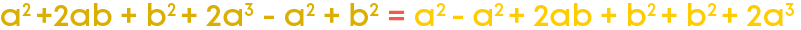Add the like terms and simplify:

a2 – a2 + 2ab + b2 + b2 + 2a3

0 + 2ab + 2b2 + 2a3

2ab + 2b2 + 2a3

We know that a term can be subtracted from a like term to give a single term. But, unlike terms cannot be subtracted the way like terms do. So, when we subtract algebraic expressions, only like terms get subtracted to give single terms.

To subtract an algebraic expression from another,

• First, write the algebraic expression from which it is to be subtracted, followed by a minus sign (-) and then the expression to be subtracted.
• Change the sign of the terms of the second expression.
• Then, arrange the like terms together.
• Subtract the like terms accordingly, to simplify.

For example, let us subtract the algebraic expression 2a3 – a2 + b2 from a2 + 2ab + b2

Write the algebraic expression from which it is to be subtracted, followed by a minus sign (-) and then the expression to be subtracted.

a2 + 2ab + b2 – (2a3 – a2 + b2)

Remove the brackets by changing the signs of the terms of the second expression.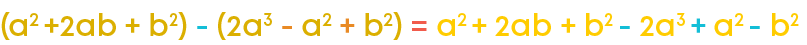Arrange the like terms together: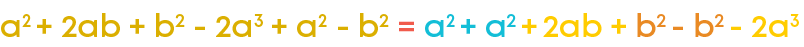Simplify:

a2 + a2 + 2ab + b2 – b2 - 2a3

= 2a2 + 2ab + 0 - 2a3

= 2a2 + 2ab - 2a3

We know that like terms can be added/subtracted by adding/subtracting their numerical coefficients. So, if an algebraic expression has like terms, it can be simplified by adding/subtracting according to the terms given in the expression.

#### Value Of An Expression

The value of an expression is the value obtained by substituting the value of the variable. Every algebraic expression has a value at a given value of the variable.

Let us learn how to find the value of an expression.

Find the value of the expression 3x + 2 at x = 2

We find the value of an expression for a given value of the variable by substituting the value in the expression.

So, substitute x = 2 in the expression

3x + 2

= 3 × 2 + 2

= 6 + 2

= 8

Hence, the value of the expression 3x + 2 at x = 2 is 8.

#### Applications Of Algebraic Expressions

We know how to find the area of a square. By multiplying the length of each side of a square by itself we get its area. If we consider the length of each side of a square to be ‘a’ unit, then what will be its area?Area = a2 is the formula to find the area of a square. By substituting the length of a given square in place of ‘a’, we get the area of the given square.

For example, the area of a square where each side measures 2 units is obtained by substituting 2 in the formula:

Area = a2 = 22 = 4

The area of the square is 4 sq. units. Here, a2 is an algebraic expression. Thus, an algebraic expression is used as a formula.

Similarly, area and perimeter of other geometrical figures are also represented using algebraic expressions which are used as formulae.

We know that for any natural number, we get its next natural number by adding 1 to it.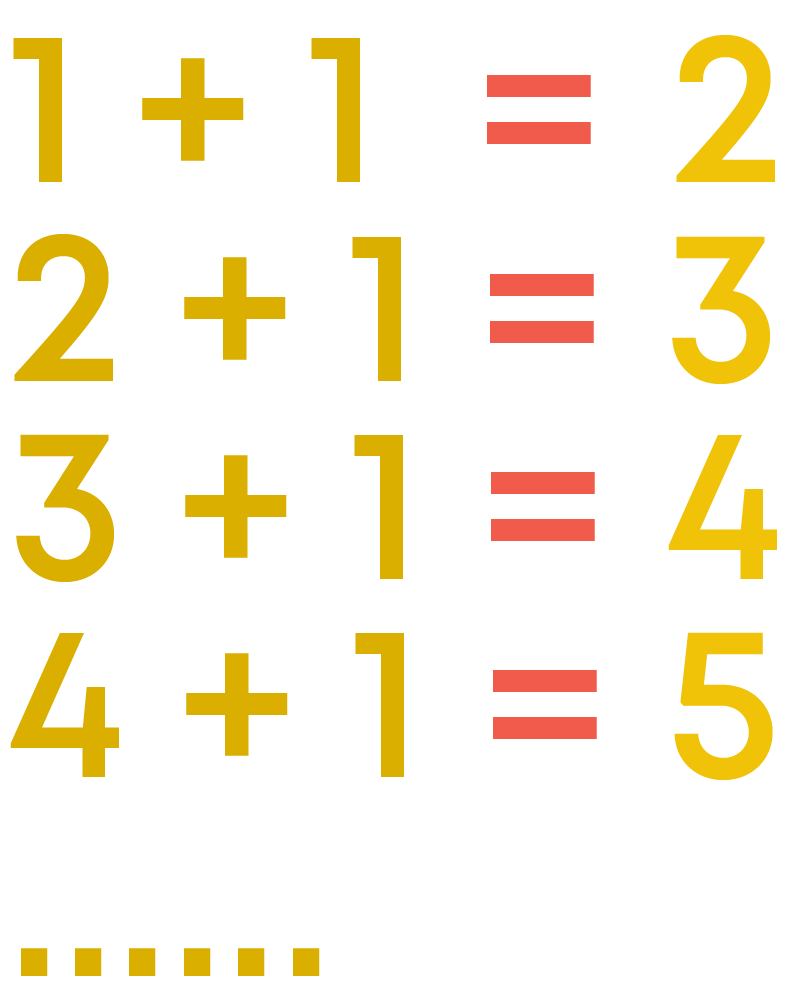So, in general, for a natural number ‘n’, its next natural number is given by n + 1. By substituting any natural number in place of ‘n’, we get its next natural number.

So, here n + 1 is used as a rule to find the next natural number. Thus, algebraic expressions are used to form arithmetic rules. Algebraic expressions are also used to represent a pictorial pattern. The relation between the number of objects and their position is established in terms of a variable by observing the pattern.

We know that the successor of a number is obtained by adding 1 to the number. So, the expression (n + 1) can be used as a rule. The successor of a particular number is obtained by substituting the number in place of ‘n’ in the expression n + 1. Similarly, we have many other sequences of numbers which follow some pattern. For example, the sequence of odd numbers, the sequence of even numbers, etc.This is the sequence of odd numbers. Which number does it start with? It starts with 1. Let us form an algebraic expression for the sequence of odd numbers.

First, tabulate the numbers and their positions to observe the pattern: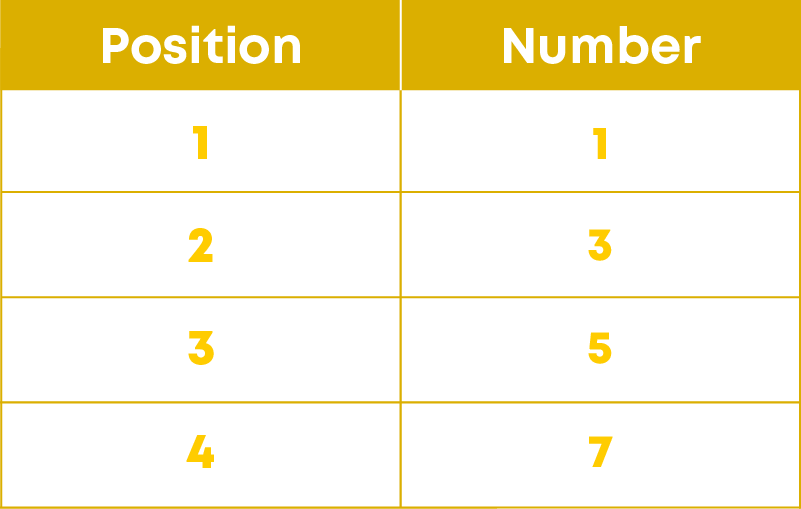Now, find the common relation between each number and its position. Each number is 1 less than twice its position.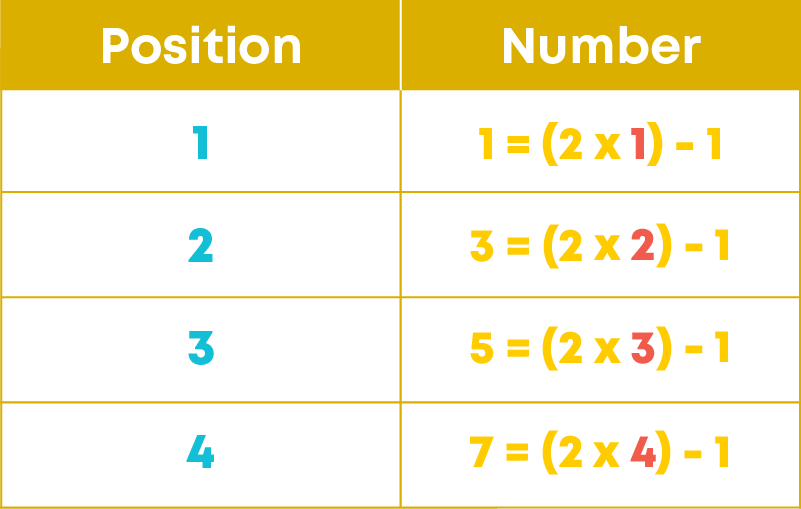So, in general, the number in the nth position can be written as 2n – 1.

In a similar way, we can write an algebraic expression for each sequence of numbers following a pattern by observing the common relation between each number and its position.

We know that algebraic expressions can be used to represent pictorial patterns and number patterns. Can they be used for geometrical patterns also? Consider this example:

ABCDEF is a hexagon and AD is its diagonal.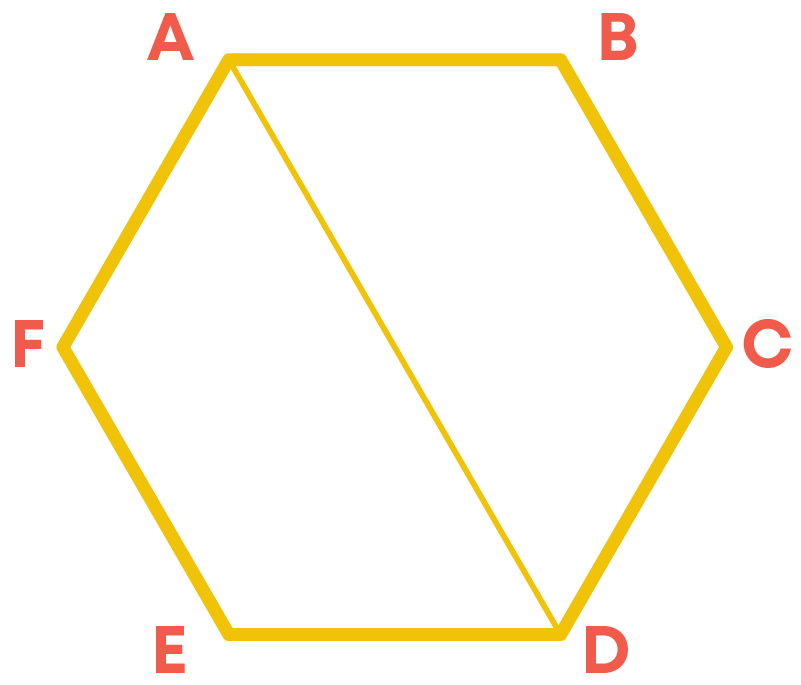Which two vertices does it join? A and D. How many more diagonals can be drawn from the vertex A? 2 more, one to C and another to E.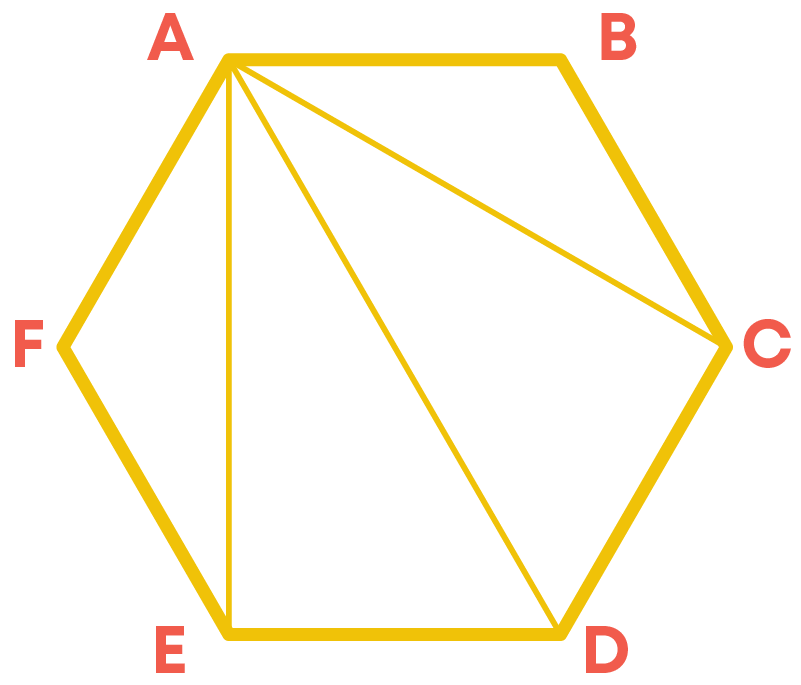So, in total, from the vertex A, 3 diagonals can be drawn. Is it same for other vertices too? Yes, from the other vertices as well, 3 diagonals can be drawn.Now, let us consider other polygons.Observe the diagonals of these polygons. Now, let us tabulate the number of sides of the polygons and the number of diagonals from its each vertex.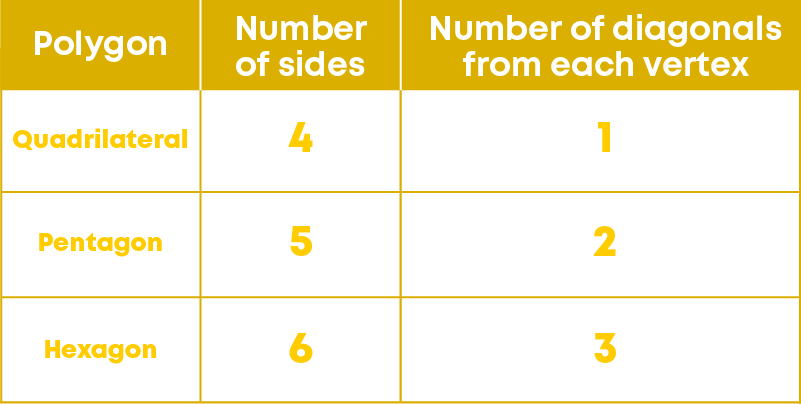Can you find the relation between the number of sides and the number of diagonals from each vertex? We observe that for all the polygons,

Number of diagonals from each vertex = Number of Sides – 3

So, in general, if ‘n’ is the number of sides of a polygon then the number of diagonals from each vertex is given by the expression ‘n – 3’.

#### Common Errors

The following are topics in which students make common mistakes when dealing with algebraic expressions:

• 1. Constants
• 2. Identifying terms of an expression
• 3. Numerical coefficient of a term
• 4. Addition/subtraction of like terms

#### Constants

Each constant is an algebraic expression, more specifically a monomial.

-3 is a constant.

-3 can be written as -3x0 since x0 = 1.

So, -3 is an algebraic expression.

Since it has only one term, it is a monomial.

#### Identifying Terms Of An Expression

While writing terms of an algebraic expression, the sign also should be considered.

Example:

The terms of the expression x3 + 2x2 – 5x – 4 are x3, 2x2, -5x, and -4.

#### Numerical Coefficient Of A Term

If no number is written with a combination of variables in a term, then the numerical coefficient of the term is 1.

Example:

The numerical coefficient of the term xy2 is 1.

While adding (or subtracting) terms with variables having exponents greater than 1, add (or subtract) only the coefficients. Do not add (or subtract) the exponents.

Example:

4p3 + 7p3 = (4 + 7)p3 = 11p3

#### Conclusion

You learnt about algebraic expressions and addition/subtraction of such expressions. Can you solve this riddle?

Observe the pyramid below and solve for X: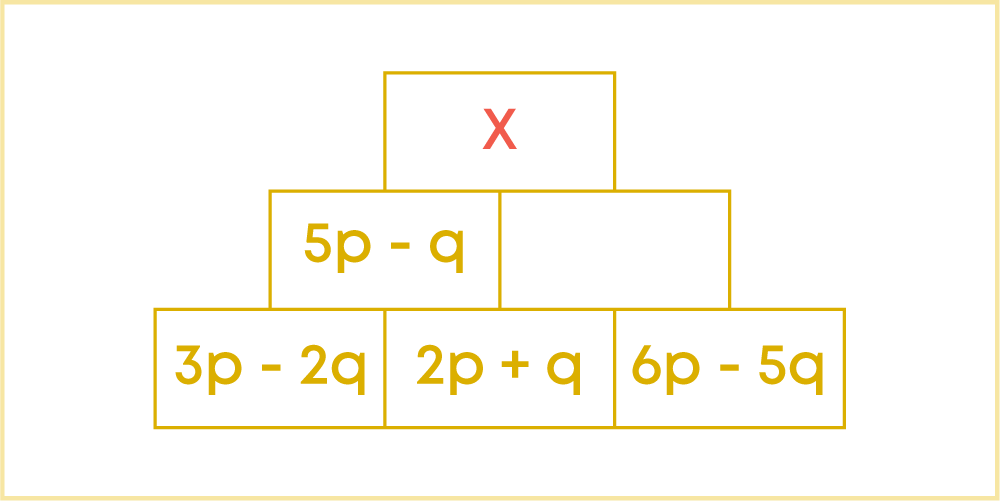#### Arpana

Author

Arpana is an education specialist with years of teaching Math and developing content. She previously worked as a freelance content developer, developing lesson plans for a reputed publisher of text books and content for various educational companies. She is an enthusiastic teacher who has taught students from India, the UK, and New Zealand.